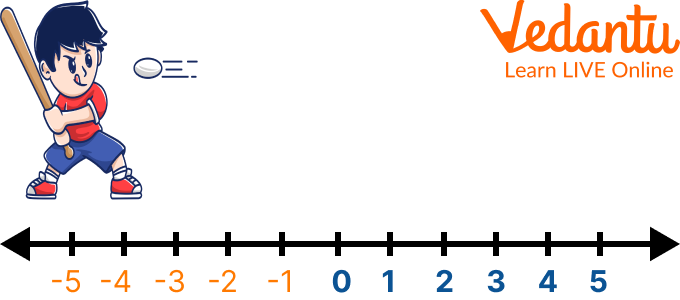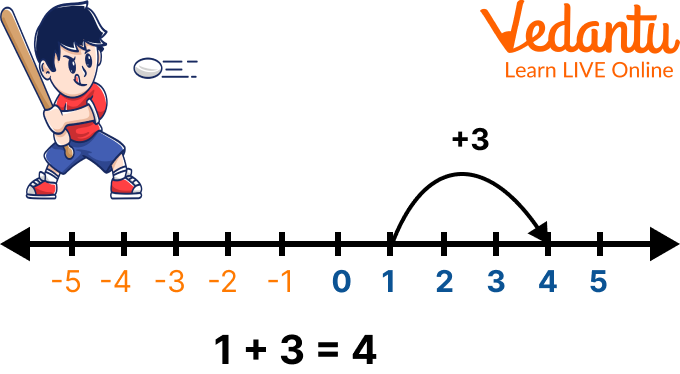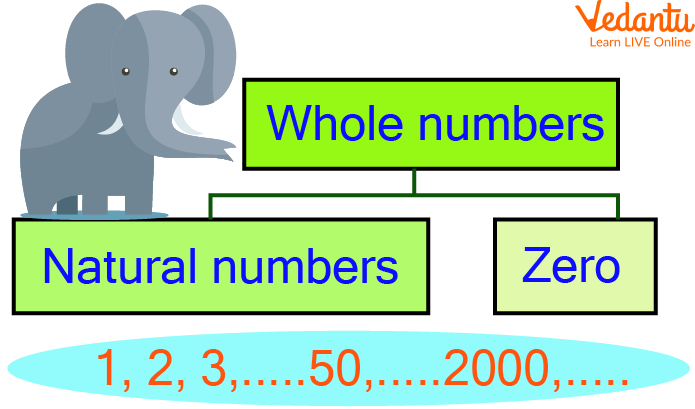Courses
Courses for Kids
Free study material
Free LIVE classes
More

# Whole Numbers on the Number LineLIVE
Join Vedantu’s FREE Mastercalss

## Overview of the Whole Numbers

What do we call the set of natural numbers or counting numbers which include zero? The set of natural numbers or counting numbers that include zero is called whole numbers. These are represented by writing, N + {0}. Whole numbers serve as the pillar for learning the countings. In the writing below, children would understand the whole numbers, unknown facts about them, and the representation of whole numbers on the number line. So, let us begin with learning about this easiest and most interesting topic.

## What Do You Mean by Whole Number?

All natural numbers and 0 are included in the category of whole numbers. In simple words, whole numbers are a set of numbers without fractions, decimals, or even negative integers. Whole numbers include counting numerals along with zero. The English alphabet W is used to denote the set of whole numbers. The bundle of whole numbers is represented as:

W = {0, 1, 2, 3, 4, ……}.

## Whole Numbers on the Number Line

To represent the whole numbers on the number line, one needs to follow some steps, which are given below:

• Draw a straight line of any length, called a number line.

• Mark all the positive integers along with 0 excluding the negative integers

• The set of numbers being shown on the number line represents the whole numbers on the number line(Blue coloured)Showing the Representation of Whole Numbers on the Number Line

## Addition of Whole Numbers on the Number Line

Follow the given series of steps for the addition of whole numbers on the number line:

• Assume any of the given numbers as the starting point on the number line

• Mark the point on the number line.

• Now, jump as many units as equivalent to the other given number towards the right side from the starting point.

• Mark the final point where you have reached after jumping.

This point gives the addition of two whole numbers on the number line.Addition of Two Whole Numbers on the Number Line

## Facts About the Whole Numbers

Some facts about the whole numbers are discussed underneath:

• Whole numbers make up all natural numbers.

• Zero along with all the positive integers forms the whole numbers.

• Real numbers consist of all the whole numbers along with other numbers.

• Zero is the smallest whole number.

• Whole numbers are not available in form of fractions.

##Showing the Whole Numbers

## Q 1. Add the whole numbers 5 and 7 using the number line.

Ans: Follow the given steps for performing the addition of whole numbers on the number line:

• Assume any of the given numbers as the starting point on the number line, say 5

• Mark the point 5 on the number line

• Now, jump as many units as equivalent to the other given number, i.e. 7 towards the right side from the starting point, 5

• Mark the final point, where you have reached i.e. 12

This point i.e. 12 gives the addition of two whole numbers, 5 and 7 on the number line.Showing the Addition of Whole Numbers on the Number Line

Q 1. Add the whole numbers 4 and 5 using the number line.

Ans: Follow the given steps for performing the addition of whole numbers on the number line:

Assume any of the given numbers as the starting point on the number line, say 4

Mark the point 4 on the number line

Now, jump as many units as equivalent to the other given number, i.e. 5 towards the right side from the starting point, 4

Mark the final point, where you have reached i.e. 9

This point i.e. 9 gives the addition of two whole numbers, 4 and 5 on the number line.Showing the Addition of Whole Numbers on the Number Line

## {1, 2, 3.5, -3, \$\dfrac{5}{2}\$, -7, 8}.

Ans. The whole numbers from the above set are 1, 2, and 8.

## Q 3. Add the given whole numbers by using the real line or number line.

1. 2 and 4 (Ans: 6)

2. 6 and 3 (Ans: 9)

## Summary

Summing up here with the topic of the whole numbers. This article covers everything about whole numbers, starting from what do you mean by whole numbers to the representation of whole numbers on the number line. Every topic discussed in the article is presented in a simple language and to-the-point format. Some solved examples along with some practice problems are being assigned to students for a better understanding of the concepts. Sense autonomous to ask your doubts in the comments below.

Last updated date: 23rd Sep 2023
Total views: 81k
Views today: 0.81k

## FAQs on Whole Numbers on the Number Line

1. What do we call the whole numbers that include negative numbers?

The whole numbers which also include negative numbers are called integers. Generally, these are represented using the English letter Z. The set of integers is given by writing, Z = { ……., -3, -2, -1, 0, 1, 2, 3, ……..}. Integers are called the superset of whole numbers and natural numbers while the subset of rational numbers and real numbers.

2. What is the definition of a number line?

The definition of a number line states that the number line is a straight horizontal line which is generally used to locate the real numbers, that includes all natural numbers, whole numbers, integers, and rational and irrational numbers. Sometimes, it is also used to find the position of a particular given point.

3. Name the properties satisfied by the whole numbers.

The properties satisfied by the whole numbers are:

• Closure Property

• Commutative Property

• Associative Property